# Construct all possible BSTs with keys 1 to N

In this article, we are going to see how many unique Binary Search trees can be constructed with keys 1 to N?
Submitted by Radib Kar, on October 12, 2020

In this article, we are going to see how many unique binary search trees are possible with key values 1 to n?

For example, with keys 1 to 3(n =3), below are the unique BSTs possible,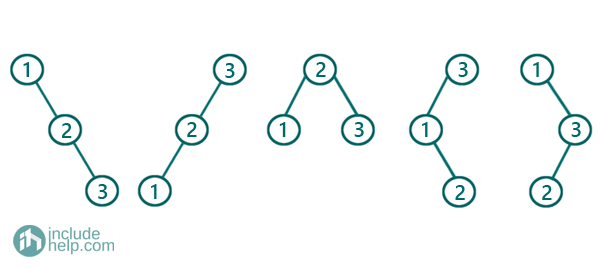As we can see, there are five different Binary search Trees created with keys 1 to 3. There we can see each of the keys has got an opportunity to be the root and then we have constructed picking up possible keys for the left subtree and the right subtree subsequently.

For example,

Say,

2 be our root

Then what are the options to pick as the root of its left subtree?

There is only one that is 1

And

What are the options to pick as the root of its right subtree?

There is only one that is 3

So, only one tree is possible with root 2 and that is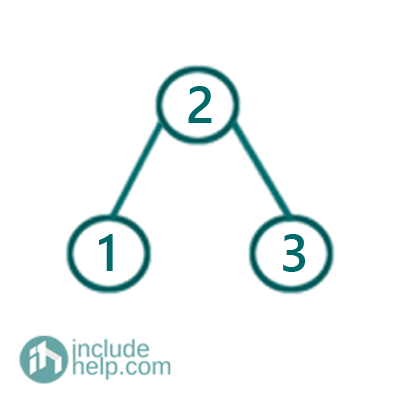Now, say we pick 3 as our root

So for the right subtree, we have no options and that has to be NULL

But for the left subtree, we have now two option to choose the root

We can either choose 2 as root or choose 1 as root

So if we choose 2 as root then the right subtree of 2 has to be NULL and the left subtree of 2 has to be 1. So the tree will be like below: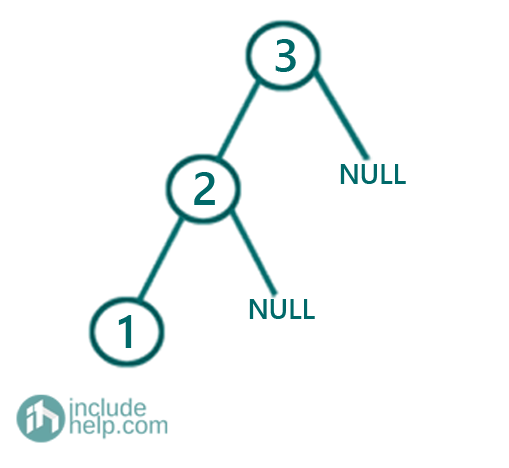If we choose 1 as the root of left subtree, then the left subtree of 1 has to be NULL and the right subtree has to be 2. So the tree would be like below: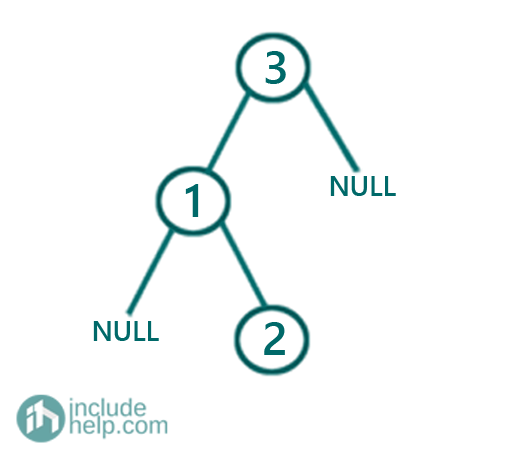What how can we generalize?

Let's say

We pick up a key k to be the root of our BST

So what is the range of options for left subtree root is [1, k-1] and range of options for right subtree root is [k+1, n]

Let's say we can have x left subtrees if we construct recursively out of those keys [1, k-1] & we can have y right subtrees if we construct recursively out of those keys [k+1, n] keys

No number of unique trees would be x*y where k is the root

So basically

```    For k = 1 to n:
Count number of left subtrees possible with
keys [1,k-1] recursively, say, the count is x
Count number of left subtrees possible with
keys [k+1, n] recursively, say,  the count is y
Total BST possible with root as k is x*y
End for
```

So basically the total number of unique BST count would be: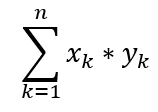Where,
xk=number of left subtrees ,yk=number of right subtrees when k is the root

Now, here we have to construct the trees too. Say the recursive function to create all unique BST is buildBst  which takes the range as an argument and returns all the trees(roots).

1. Create a list to store the trees, say arr
2. ```If (left>right):
Add NULL to the list and return since no BST is possible
End If
```
For key k= left to right
1. Find the list of left subtrees recursively which can be found by calling buildBST(left,k-1);
2. Find the list of left subtrees recursively which can be found by calling buildBST(k+1, right)
Now for each combination from left tree list and right tree list, create the BSTs and store that into list arr. That's illustrated below: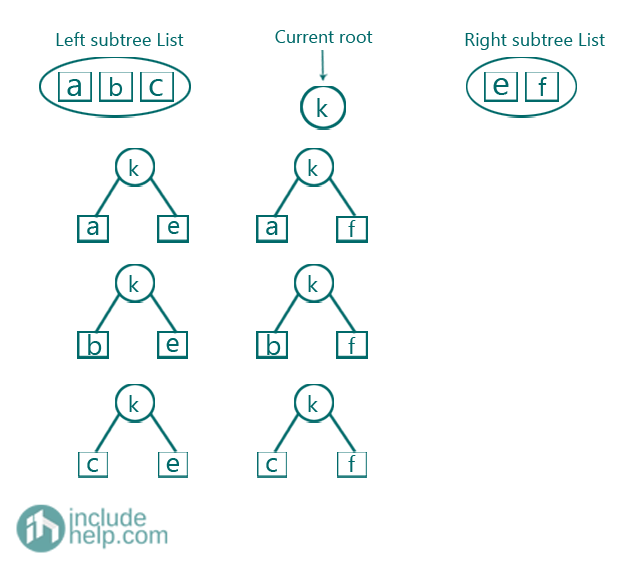So we can see that the left subtree list has three possible BSTs namely a, b, c & the right subtree list has 2 possible BST namely e & f. So we can have total 3*2=6 combinations. ALL the combinations are valid BST because all keys in any left subtrees are less than root and all keys in any right subtrees are greater than root and they are all BSTs.
3. return arr;
END FUNCTION

Below is the C++ implementation of the above idea.

```#include <bits/stdc++.h>
using namespace std;

// tree node is defined
class TreeNode {
public:
int val;
TreeNode* left;
TreeNode* right;
TreeNode(int data)
{
val = data;
left = NULL;
right = NULL;
}
};

//preorder traversal
void preorder(TreeNode* root)
{
if (!root)
return;
cout << root->val << " ";
preorder(root->left);
preorder(root->right);
}

//recursive function to construct all possible BSTs
vector<TreeNode*> buildBST(int left, int right)
{
//vector to store all BSts
vector<TreeNode*> arr;
//base case
if (left > right) {
arr.push_back(NULL);
return arr;
}
//for eachj possible key in b/w [left,right], make that as root
for (int i = left; i <= right; i++) {
//find list of possible left subtrees recursively
vector<TreeNode*> leftSubtrees = buildBST(left, i - 1);
//find list of possible right subtrees recursively
vector<TreeNode*> rightSubtrees = buildBST(i + 1, right);
//for all possible combinations build the BSTs
for (auto it : leftSubtrees) {
for (auto ij : rightSubtrees) {
TreeNode* root = new TreeNode(i);
root->left = it;
root->right = ij;
arr.push_back(root);
}
}
}

return arr;
}

vector<TreeNode*> generateUniqueBSTs(int n)
{
vector<TreeNode*> vv;
if (n == 0)
return vv;

vector<TreeNode*> res = buildBST(1, n);

return res;
}

int main()
{
int n;

cout << "Enter n:\n";
cin >> n;

int countOfTrees = 0;

vector<TreeNode*> arr = generateUniqueBSTs(n);

countOfTrees = arr.size();

cout << "Number of possible unique BSTs with keys 1 to " << n << " is: " << countOfTrees << endl;

cout << "preorder traversal of all possible trees are:\n";
for (auto ik : arr) {
preorder(ik);
cout << "\n";
}

return 0;
}
```

Output:

```Enter n:
3
Number of possible unique BSTs with keys 1 to 3 is: 5
preorder traversal of all possible trees are:
1 2 3
1 3 2
2 1 3
3 1 2
3 2 1
```

Since the dry run would be very exhaustive, I am leaving that part up to you and you can comment below if you find any difficulty doing the dry run your own.

But it's not the end. I have something exciting to come up. If we were asked do we need to do all these recursions? The number of unique Binary Search trees are Catalan Numbers. Catalan numbers are a series of numbers and can be described by the formula: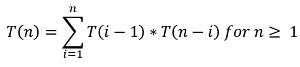The recursion is something we have already discussed in terms of the left subtree list & the right subtree list. So, we can use DP to memorize this recursion and can compute that too. Also, Catalan numbers can be expressed as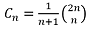and we can use this formula to generate number of unique BSTs possible. But it won't return all trees and will give you numbers only. So, though it's handy to know I would recommend you to learn the solution first and implement that to see the beauty of recursion.